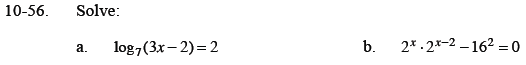Home > CCA2 > Chapter 10 > Lesson 10.1.3 > Problem10-56

10-56.
1. Solve: Homework Help ✎

1. log7(3x − 2) = 2

2. 2x ⋅ 2x−2 − 162 = 0Rewrite the equation in exponential form.

72 = 3x − 2

22x − 2 = 162

22x − 2 = (24)2